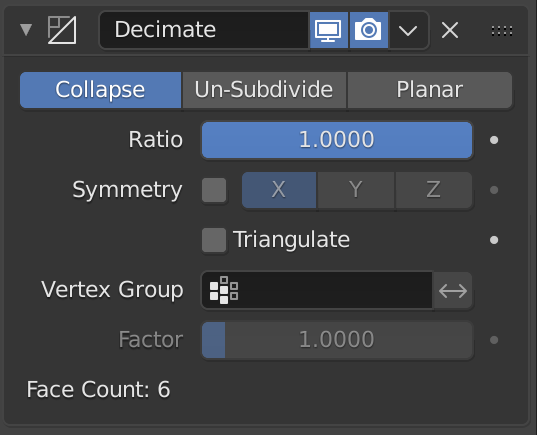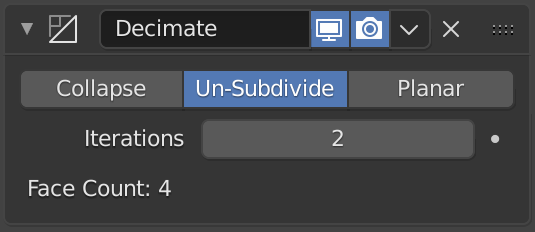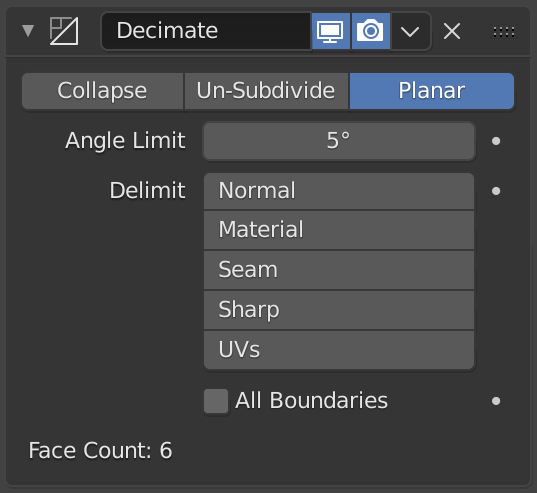# Decimar¶

The Decimate modifier allows you to reduce the vertex/face count of a mesh with minimal shape changes.

This is not usually used on meshes which have been created by modeling carefully and economically (where all vertices and faces are necessary to correctly define the shape). But if the mesh is the result of complex modeling, sculpting and/or applied Subdivision Surface/ Multiresolution modifiers, the Decimate one can be used to reduce the polygon count for a performance increase, or simply remove unnecessary vertices and edges.

Unlike the majority of existing modifiers, this one does not allow you to visualize your changes in Edit Mode.

The modifier displays the number of remaining faces as a result of the Decimate modifier.

## Opções¶

### Mode¶

#### Recolher¶The Decimate modifier in Collapse mode.

Merges vertices together progressively, taking the shape of the mesh into account.

Proporção (Taxa)

The ratio of faces to keep after decimation.

• On 1.0: the mesh is unchanged.

• On 0.5: edges have been collapsed such that half the number of faces remain (see note below).

• On 0.0: all faces have been removed.

Nota

Although the Ratio is directly proportional to the number of remaining faces, triangles are used when calculating the ratio.

This means that if your mesh contains quads or other polygons, the number of remaining faces will be larger than expected, because those will remain unchanged if their edges are not collapsed.

This is only true if the Triangulate option is disabled.

Simetrizar

Maintains symmetry on a single axis.

Triangular

Keeps any resulting triangulated geometry from the decimation process.

Grupo de vértices

A vertex group that controls what parts of the mesh are decimated.

Fator

The amount of influence the Vertex Group has on the decimation.

#### (De-)Subdividir¶The Decimate modifier in Un-Subdivide mode.

It can be thought of as the reverse of subdivide. It attempts to remove edges that were the result of a subdivide operation. It is intended for meshes with a mainly grid-based topology (without giving uneven geometry). If additional editing has been done after the subdivide operation, the results may be unexpected.

Interações

The number of times to perform the un-subdivide operation. Two iterations is the same as one subdivide operation, so you will usually want to use even numbers.

#### Plainar¶The Decimate modifier in Planar mode.

It reduces details on forms comprised of mainly flat surfaces.

Limite angular

Dissolve geometry which form angles (between surfaces) higher than this setting.

Delimitar

Prevent dissolving geometry in certain places.

Normais

Does not dissolve edges on the borders of areas where the face normals are reversed.

Materiais

Does not dissolve edges on the borders of where different materials are assigned.

Costuras

Does not dissolve edges marked as seams.

Todas as margens

When enabled, all vertices along the boundaries of faces are dissolved. This can give better results when using a high Angle Limit.